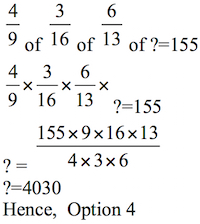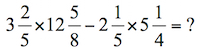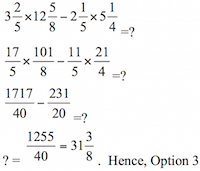# Sample Aptitude Questions of Sony

1. 4/9 of 3/16 of 6/13 of ? = 155
1. 4420
2. 4240
3. 4320
4. 4030
5. 41202. 156.25 × 12.4 + 1.8 × 52.5 = ? – 175.85
1. 2124.5
2. 2212.6
3. 2207.85
4. 2684.8
5. 2624.4

156.25×12.4+1.8×52.5=?-175.85
1937.5+94.5=?-175.85
2032=?-175.85
?=2207.85
Hence, Option C.
1.1. 35 1/5
2. 35 5/8
3. 31 3/8
4. 32 2/5
5. 33 1/8DIRECTIONS for the questions 4 to 10 : Solve the following question and mark the best possible option.
2. S mixed 36 kg of sugar @ Rs. 45/- per kg and 24 kg of sugar @ Rs. 48/- per kg and sold the mixture as to earn 20% profit. At what rate per kg must he sell the sugar.
1. Rs. 52.56
2. Rs. 52.42
3. Rs. 52.36
4. Rs. 55.44
5. Rs. 54.25

Total cost price=36×45+24×48=2772/-
Average cost price per kg = 2772/60 = Rs. 46.2
S.P=Rs 55.44 per kg( @20% profit)
3. The length of a rectangle exceeds its breadth by 6m. Length of the rectangle is equal to the side of a square whose area is 729 sq. m. What is the perimeter of the rectangle? (in m)
1. 96
2. 108
3. 92
4. 88
5. 84

Let ‘l’ be the length, ‘b’ be the breadth of the rectangle.
Length of the rectangle =Side of the square of area 729m2
∴ Length of the rectangle =27m. A.T.Q. l-b=6
⇒ b = l - 6 = 21m ⇒ l = 27m
Perimeter of the rectangle = 2 (l + b) = 2 (27 + 21) = 96m
Hence, Option A.
4. Raghuvir purchased 10 calculators and 16 watches for Rs. 56,100/- and sold them so as to earn an overall profit of 20%. At what total price should he sell 15 calculators and 24 watches together so as to earn the same percent profit?
1. Rs. 1,00,980/-
2. Rs. 1,16,176/-
3. Rs. 1,21,176/-
4. Rs. 1,00,660/-
5. Rs. 1,24,132/-

CP of 10 calculators , 16 watches =Rs.56100/-
CP of 15 calculators, 24 watches=1.5×56100=84150/-
Profit=20%
∴S.P. of 15 calculators, 24 watches =1.2×84150 = Rs. 100,980/-
Hence, Option A.
5. Some chocolates were distributed among 4 friends A, B, C and D such that respective ratio of chocolates received by A to chocolates received by C was 7:9. B received 29 more chocolates than A and D received 33 more chocolates than C. If B received 15 more chocolates than C, how many chocolates did D receive?
1. 84
2. 96
3. 72
4. 99
5. 87

A=7x, C=9x, B=7x+29, D=9x+33
A.T.Q. 29 + 7x =15+9x
⇒ x = 7
Hence, Option B.
6. Abhijit invested an amount with company X for two years @ simple interest rate 15 p.c.p.a. The entire amount obtained from company X after two years he invested with company Y @ compound interest rate 12 p.c.p.a for two years. If the amount finally received by him was Rs. 81,536. Find the money invested by abhijit in company X.
1. Rs. 65,000/-
2. Rs. 60,000/-
3. Rs. 56,000/-
4. Rs. 50,000/-
5. Rs. 45,000/-

Let ‘y’ be the invested amount
A.T.Q.
1.3y(1.12)2=81536
On Solving y=Rs.50,000/-
∴Hence, Option D.
7. Average weight of 40 students in a class is 55 kg. Six of them whose average weight is 52 kg left the class and another set of six students, whose average weight is 42kg joined the class. What is the next average weight of the class (in kg?)
1. 54.25
2. 52.75
3. 53.5
4. 54
5. 53

New Average weight = (40 * 55 - 60)/40 = 53.5 kg
Hence, Option C.
8. The ratio of roses and lilies in a garden is 3: 2 respectively. The average number of roses & lilies is 180. What is the number of lilies in the garden?
1. 144
2. 360
3. 182
4. 216
5. None